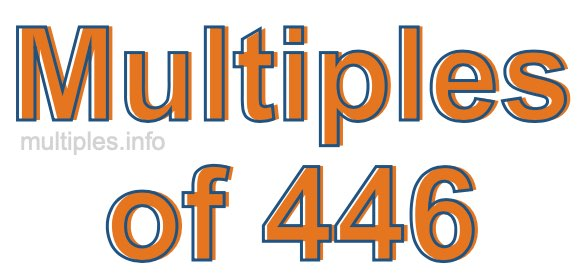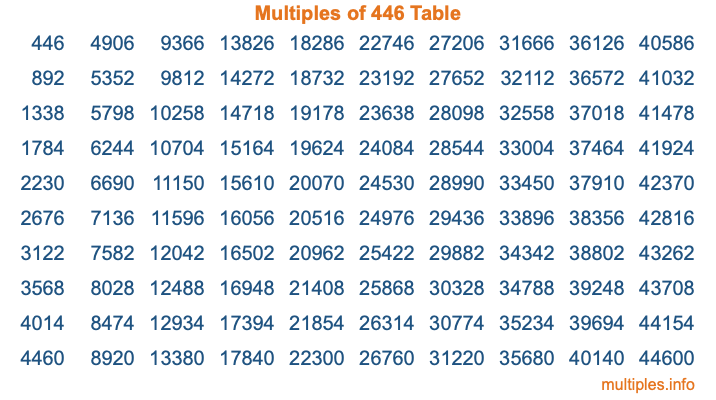Multiples of 446Welcome to the Multiples of 446 page. Here we will first teach you everything you will ever need to know about the multiples of 446, and then give you a study guide summary of everything we taught you to make sure you remember it all. Use this page to look up facts and learn information about the multiples of 446. This page will make you a multiples of four hundred forty-six expert!

Definition of Multiples of 446
Multiples of 446 are all the numbers that when divided by 446 equal an integer. Each of the multiples of 446 are called a multiple. A multiple of 446 is created by multiplying 446 by an integer.

Therefore, to create a list of multiples of 446, you start with 1 multiplied by 446, then 2 multiplied by 446, then 3 multiplied by 446, and so on for as long as you want. Thus, the list of the first five multiples of 446 is 446, 892, 1338, 1784, and 2230. To see a larger list of multiples of 446, see the printable image of Multiples of 446 further down on this page. We also have a category where you can choose any nth multiple of 446.

Multiples of 446 Checker
The Multiples of 446 Checker below checks to see if any number of your choice is a multiple of 446. In other words, it checks to see if there is any number (integer) that when multiplied by 446 will equal your number. To do that, we divide your number by 446. If the the quotient is an integer, then your number is a multiple of 446.

Is  a multiple of 446?

Least Common Multiple of 446 and ...
A Least Common Multiple (LCM) is the lowest multiple that two or more numbers have in common. This is also called the smallest common multiple or lowest common multiple and is useful to know when you are adding our subtracting fractions. Enter one or more numbers below (446 is already entered) to find the LCM.

Check out our LCM Calculator if you need more details about the Least Common Multiple or if you need the LCM for different numbers for adding and subtraction fractions.

nth Multiple of 446
As we stated above, 446 is the first multiple of 446, 892 is the second multiple of 446, 1338 is the third multiple of 446, and so on. Enter a number below to find the nth multiple of 446.

th multiple of 446

Multiples of 446 vs Factors of 446
446 is a multiple of 446 and a factor of 446, but that is where the similarities end. All postive multiples of 446 are 446 or greater than 446. All positive factors of 446 are 446 or less than 446.

Below is the beginning list of multiples of 446 and the factors of 446 so you can compare:

Multiples of 446: 446, 892, 1338, 1784, 2230, etc.

Factors of 446: 1, 2, 223, 446

As you can see, the multiples of 446 are all the numbers that you can divide by 446 to get a whole number. The factors of 446, on the other hand, are all the whole numbers that you can multiply by another whole number to get 446.

It's also interesting to note that if a number (x) is a factor of 446, then 446 will also be a multiple of that number (x).

Multiples of 446 vs Divisors of 446
The divisors of 446 are all the integers that 446 can be divided by evenly. Below is a list of the divisors of 446.

Divisors of 446: 1, 2, 223, 446

The interesting thing to note here is that if you take any multiple of 446 and divide it by a divisor of 446, you will see that the quotient is an integer.

Multiples of 446 Table
Below is an image of the first 100 multiples of 446 in a table. The table is in chronological order, column by column. The first column has the first ten multiples of 446, the second column has the next ten multiples of 446, and so on.The Multiples of 446 Table is also referred to as the 446 Times Table or Times Table of 446. You are welcome to print out our table for your studies.

Negative Multiples of 446
Although not often discussed or needed in math, it is worth mentioning that you can make a list of negative multiples of 446 by multiplying 446 by -1, then by -2, then by -3, and so on, to get the following list of negative multiples of 446:

-446, -892, -1338, -1784, -2230, etc.

Multiples of 446 Summary
Below is a summary of important Multiples of 446 facts that we have discussed on this page. To retain the knowledge on this page, we recommend that you read through the summary and explain to yourself or a study partner why they hold true.

There are an infinite number of multiples of 446.

A multiple of 446 divided by 446 will equal a whole number.

446 divided by a factor of 446 equals a divisor of 446.

The nth multiple of 446 is n times 446.

The largest factor of 446 is equal to the first positive multiple of 446.

446 is a multiple of every factor of 446.

446 is a multiple of 446.

A multiple of 446 divided by a divisor of 446 equals an integer.

446 divided by a divisor of 446 equals a factor of 446.

Any integer times 446 will equal a multiple of 446.

Multiples of a Number
Here you can get the multiples of another number, all with the same attention to detail as we did for multiples of 446 on this page.

Multiples of
Multiples of 447
Did you find our page about multiples of four hundred forty-six educational? Do you want more knowledge? Check out the multiples of the next number on our list!